# PSoC™ 5, 3 & 1 Forum DiscussionsLevel 2
Level 2Hi all,

Is it possible to read negative values with the ADC on the PSoC5 LP. If so how should it be configured?

I need to read values from -10mV to -1.5V.

1 SolutionLevel 9
Level 9JaRa,

One way to measure negative voltage is to use a resistor divider network as described by Aubrey Kagan:

Attached are a copy of the original paper, demo project, and Excel file to calculate resistor values. Note that there are only two binding equations and three resistor values, so the number of possible solutions is infinite. By selecting a starting values for R1,R2 and R3 it is possible to affect resulting solution. The Excel solver tends to slip into very large values (megaohms), which are not useful, to resolve this issue try to set some small starting values for R1-R3 (like 9).

Note that in order not to affect the resistor network, a Delsig_ADC is used, which has a buffer with very large input impedance. The ADC_SAR input impedance is rather small (~20k), affecting the measurement, so additional buffer Opamp is a must. Unfortunately the PSoC5 Opamp input impedance is not very high (~1M), so DelSig_ADC is preferred.

Once ADC voltage is measured, the input voltage can be calculated as:

Vin = ((RE1*RE2 + RE2*RE3 + RE1*RE3) * Vadc  -  RE1*RE3 * Vdd) / (RE2*RE3)

The calculated value depends on  the Vdd, which has to be stable for reproducible results.

/odissey1

Figure 1. Resistor network for shifting range from [-2V, +2V] into [0V, 2V] for ADC conversion. Note that ADC range is set to only half of that range [0V, 1.024V] to cover only negative portion of the input range.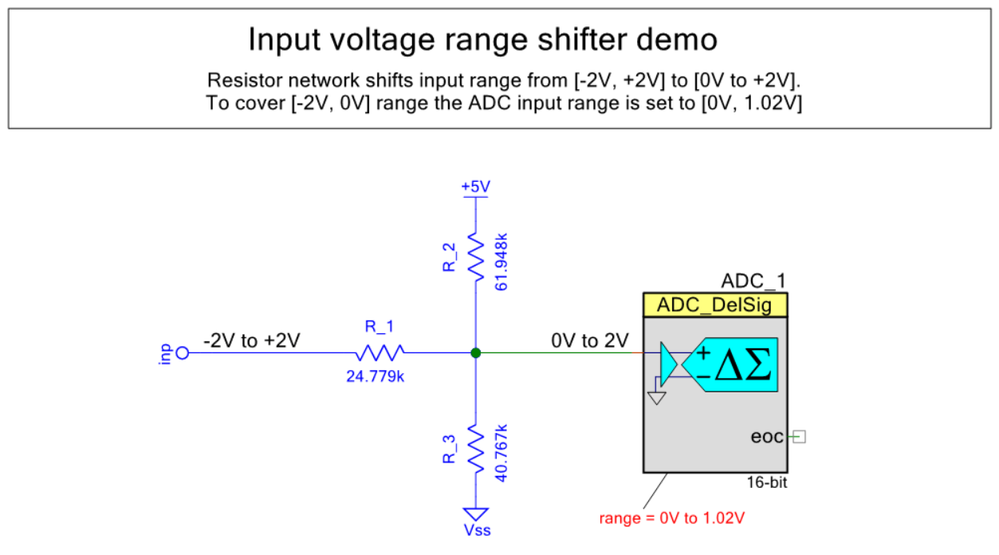Resistor network R1-R3 to shift input range from [-2V, +2V] into [0V-2V] range. Note that ADC range is set to only half range [0,V 1.02V] to cover only negative input voltages.

Figure 2. Use Data->Solver to obtain resistor values (R1,R2,R3).Using Excel spreadsheet solver to obtain R1,R2,R3

13 RepliesLevel 9
Level 9JaRa,

One way to measure negative voltage is to use a resistor divider network as described by Aubrey Kagan:

Attached are a copy of the original paper, demo project, and Excel file to calculate resistor values. Note that there are only two binding equations and three resistor values, so the number of possible solutions is infinite. By selecting a starting values for R1,R2 and R3 it is possible to affect resulting solution. The Excel solver tends to slip into very large values (megaohms), which are not useful, to resolve this issue try to set some small starting values for R1-R3 (like 9).

Note that in order not to affect the resistor network, a Delsig_ADC is used, which has a buffer with very large input impedance. The ADC_SAR input impedance is rather small (~20k), affecting the measurement, so additional buffer Opamp is a must. Unfortunately the PSoC5 Opamp input impedance is not very high (~1M), so DelSig_ADC is preferred.

Once ADC voltage is measured, the input voltage can be calculated as:

Vin = ((RE1*RE2 + RE2*RE3 + RE1*RE3) * Vadc  -  RE1*RE3 * Vdd) / (RE2*RE3)

The calculated value depends on  the Vdd, which has to be stable for reproducible results.

/odissey1

Figure 1. Resistor network for shifting range from [-2V, +2V] into [0V, 2V] for ADC conversion. Note that ADC range is set to only half of that range [0V, 1.024V] to cover only negative portion of the input range.Resistor network R1-R3 to shift input range from [-2V, +2V] into [0V-2V] range. Note that ADC range is set to only half range [0,V 1.02V] to cover only negative input voltages.

Figure 2. Use Data->Solver to obtain resistor values (R1,R2,R3).Using Excel spreadsheet solver to obtain R1,R2,R3Level 2
Level 2Interesting, can I use the power supply pin VDD as the 5V and PSoC GND?.Level 9
Level 9Sure, any Vdd and GND will do.Level 9
Level 9JaRa,

This is another way to invert input voltage range using external opamp. The advantage is that measured voltage is not affected by Opamp power lines fluctuations, and SAR_ADC can be used.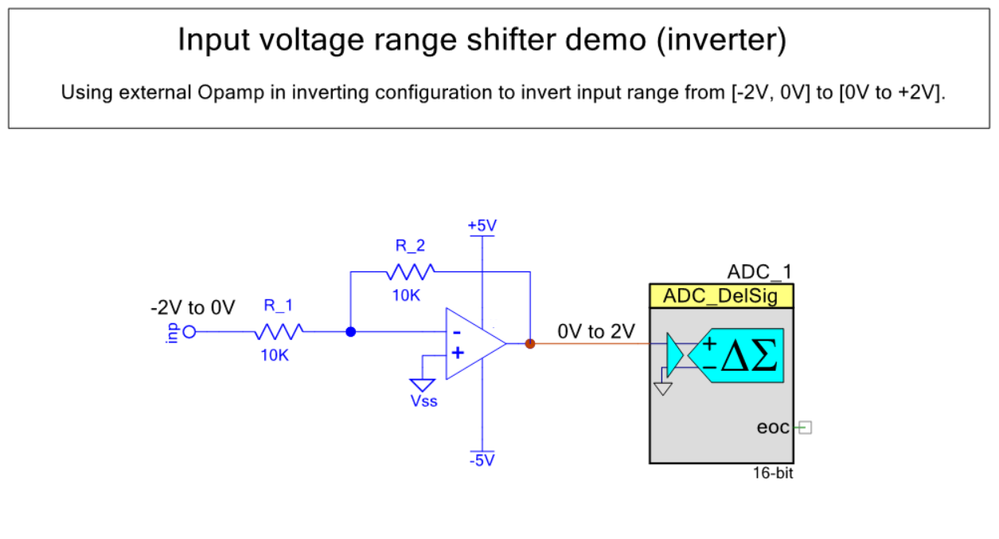Level 2
Level 2Since I have both negative and positive values, I guess this will not work?Level 9
Level 9JaRa,

If you have other requirements, it is time to post themLevel 2
Level 2Ups sorry I meant +1.5. But

I look into my data again: I have to outputs. One output goes from +400mV to -400mV. The second output goes from -100mV to -1V (negative the whole way).Level 7
Level 7you can use TIA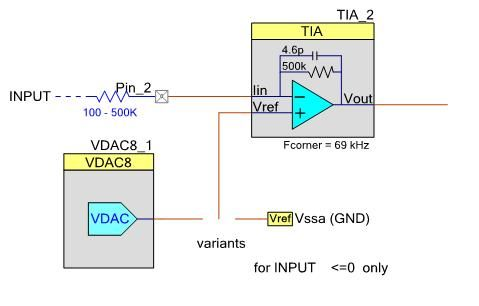Level 2
Level 2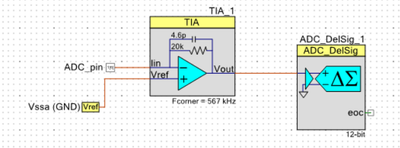I just tried this - by attachting the vdd 4.9V to the input of the TIA. It gives only ADC value of 13. What did I do wrong?Level 9
Level 9JaRa,

1. Notice 100k resistor at the input of TIA. 2. It is clearly stated that TIA schematic is for negative input only.Level 7
Level 7Check out the attached project.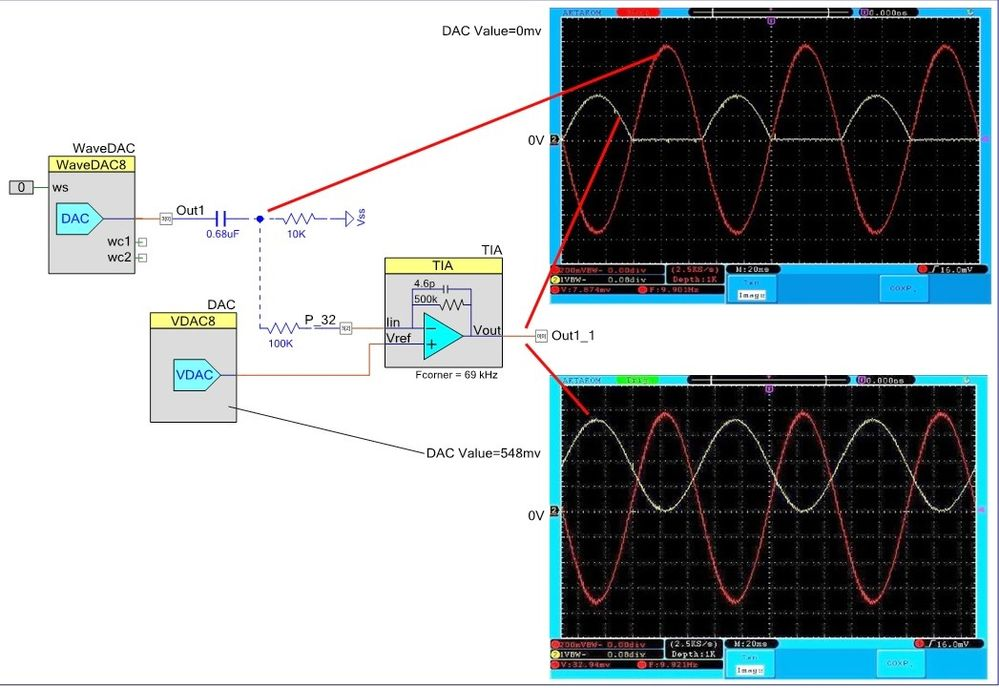Level 9
Level 9JaRa,

Yet another method to measure negative voltage is using PSoC internal Opamp in inverting configuration (Figure 1). The advantage is that only a couple of external resistors are needed and ADC_SAR can be used. Notice that though input voltage is negative, pins Pin_34 and Pin_36 voltages comply with PSoC allowed voltage range (0-5.5V). Also, the PSoC5 Opamps are not rail-to-rail, so measurements in vicinity of -0V may slightly deviate from the actual input.

Attached is a demo project using UART to output data to external plotting software (SerialPlot). The project uses a couple of custom components (SerialPlot and PSoC AnnotationLibrary) which can be found here:

/odissey1Figure 1. Project schematicFigure 2. Using SerialPlot component to output ADC data for visualizationFigure 3. Project annotation using PSoC Annotation Library v1.0. Note that USB-UART convertion is performed inside the KitProg, no external connection is necessary.Figure 4. Input signal from external signal generator. Sine Vpp=2V, offset=-1V, frequency=1Hz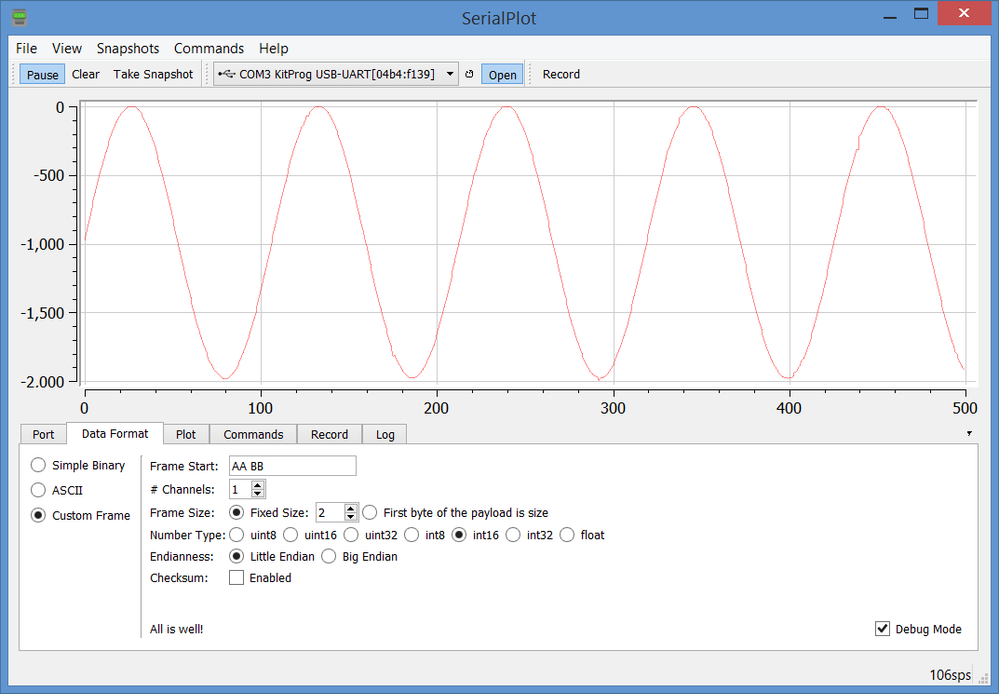Figure 5. SerialPlot output terminal window. Vertical scale (mV), data sampling rate - 100 samples/sec.Level 9
Level 9JaRa,

Attached is updated project, showing shifting ADC sampling range using Opamp. Two input ranges are shown [-0.5V, +0.5V], and [-1V, 0V], which can be achieved by simply changing the Opamp reference voltage. Opamp resistor divider is set to R1=R2=10k, but any pair of resistors of same value will do (e.g. 100k). Select resistor value depending on sensor output impedance.

/odissey1

Figure 1. DC transfer characteristics for single-supply Opamp.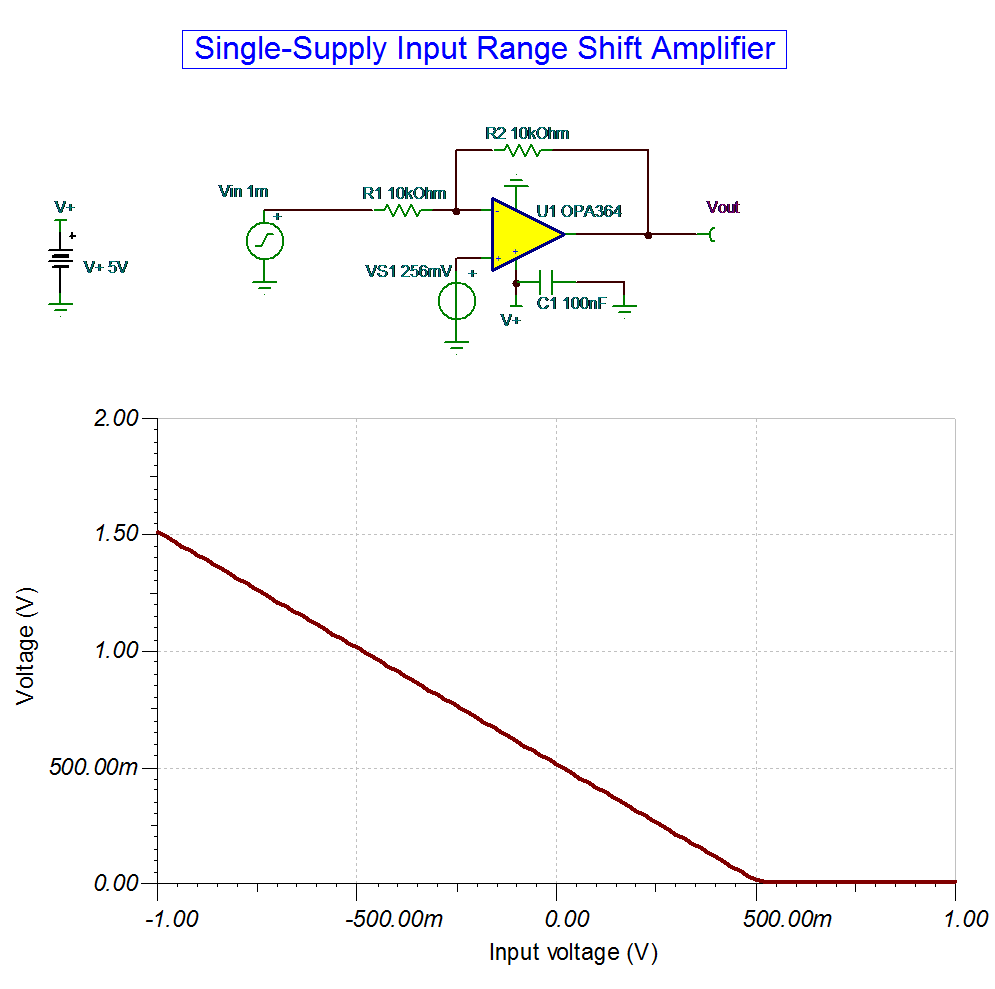Figure 2. Project schematic for [-0.5V, +0.5V] sampling range. Vref = 0.256V.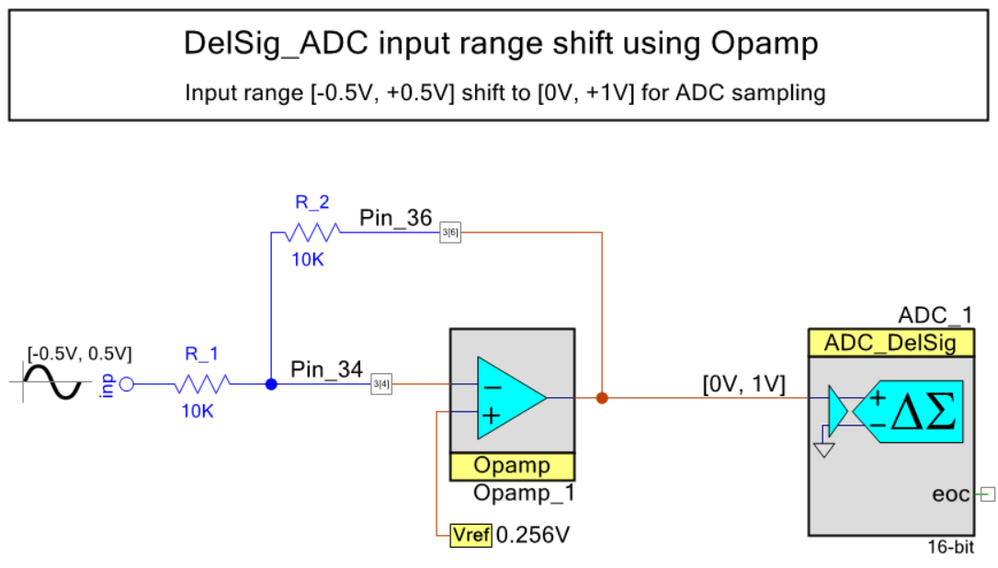Figure 3. Project annotation using PSoC Annotation Library v1.0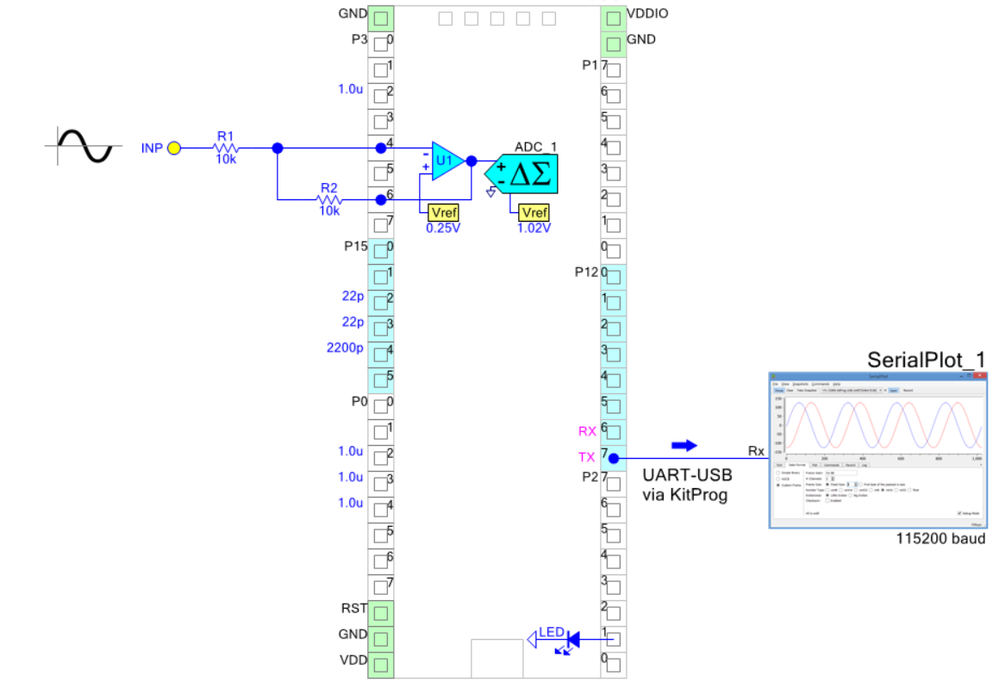Figure 4. Input signal from signal generator in range [-0.5V, +0.5V]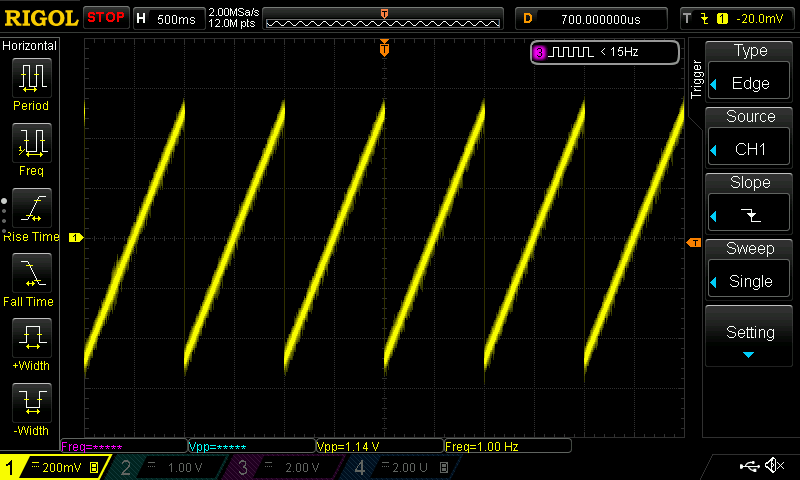Figure 5. Measured input signal (mV), plotted using SerialPlot charting software. ADC sampling rate 100Hz.Figure 6. Project schematic for [-1V, 0V] sampling range. Vref = Vssa (GND).